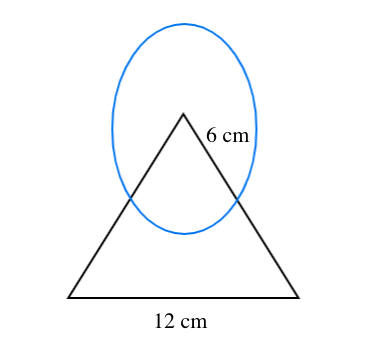Courses
Courses for Kids
Free study material
Free LIVE classes
MoreLIVE
Join Vedantu’s FREE Mastercalss

# In the figure given below find the area of the shaded region where a circular arc of radius 6 cm has been drawn with vertex O of an equilateral triangle OAB of side 12 cm as center.Verified
366.3k+ views
Hint: - Area of shaded region $=$Area of circle$+$Area of equilateral triangle$-$area of
common region.
Given data:
Radius of circle$\left( r \right) = 6m$
Side of an equilateral triangle$\left( a \right) = 12cm$
As we know area of circle$= \pi {r^2} = \dfrac{{22}}{7} \times {6^2} = \dfrac{{792}}{7}c{m^2}$
Now as we know area of equilateral triangle$= \dfrac{{\sqrt 3 }}{4}{a^2} = \dfrac{{\sqrt 3 }}{4} \times {12^2} = 36\sqrt 3 c{m^2}$
Area of common region (i.e. between circle and equilateral triangle)
$\Rightarrow \left( {\dfrac{\theta }{{{{360}^0}}}} \right)\pi {r^2}$
As we know in equilateral triangles all angles equal to${60^0}$.
$\Rightarrow \angle {\text{AOB}} = {60^0} = \theta$
Therefore area of common region$= \left( {\dfrac{\theta }{{{{360}^0}}}} \right)\pi {r^2} = \dfrac{{{{60}^0}}}{{{{360}^0}}} \times \dfrac{{22}}{7} \times {6^2} = \dfrac{{132}}{7}c{m^2}$
Therefore, the area of the shaded region$\left( A \right)$$=$Area of circle$+$Area of equilateral

triangle$-$area of the common region.
$\Rightarrow \left( A \right) = \dfrac{{792}}{7} + 36\sqrt 3 - \dfrac{{132}}{7} = \left( {\dfrac{{660}}{7} + 36\sqrt 3 } \right)c{m^2}$
So, this is the required answer.

Note: -In such types of questions always remember the formula of area of standard shapes which is
stated above, then first find out the area of circle then find out the area of triangle then find out the
area of common region, then find out the area of shaded region using the formula which is stated above
then simplify we will get the required answer.

Last updated date: 04th Oct 2023
Total views: 366.3k
Views today: 3.66k